## Kinematics Exam3 and Problem Solutions

Kinematics Exam3 and  Problem Solutions

1. As you can see from the given picture, ball is thrown horizontally with an initial velocity. Find the time of motion. (g=10m/s2)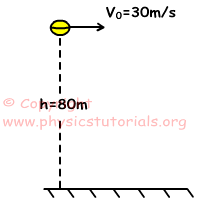Ball does projectile motion in other words it does free fall in vertical and linear motion in horizontal. Time of motion for horizontal and vertical is same. Thus in vertical;

h=1/2g.t2

80=1/2.10.t2

t=4s

2. An object hits the ground as given in the picture below. Find the initial velocity of the object.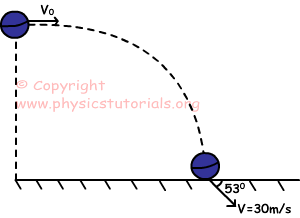Velocity of horizontal motion is constant. So;

V0=Vx=Vcos530

Vx=V0=30m/s.0,6

V0=Vx=18m/s

3. An object is thrown with an angle 370 with horizontal. If the initial velocity of the object is 50m/s, find the time of motion, maximum height it can reach, and distance in horizontal.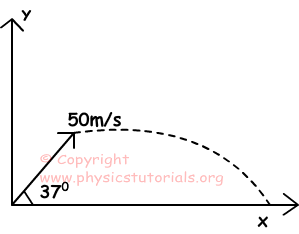V0x=V0cos530=50.0,8=40m/s

V0y=V0y.sin530=50.0,6=30m/s

a) V-V0y=0-g.t at the maximum height

t=30/10=3s

2.t=time of motion=2.3=6s

b) V0y2=hmax.2.g

hmax=302/2.10=45m

c) X=V0x.ttotal=40.6=240m

4. A balloon having 20 m/s constant velocity is rising from ground to up. When the balloon reaches 160 m height, an object is thrown horizontally with a velocity of 40m/s with respect to balloon. Find the horizontal distance travelled by the object.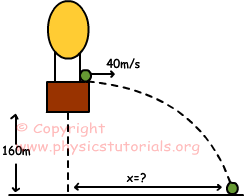Object has velocity 40m/s in horizontal, 20m/s in vertical and its height is 160m. We can find time of motion with following formula;

h=V0y.t-1/2.g.t2

-160=20.t-1/2.10.t2

t2=4t-32

(t-8).(t+8)=0

t=8s

X=V0x.t=40.8=320m.

5. Objects A and B are thrown with velocities as shown in the figure below. Find the ratio of horizontal distances taken by objects.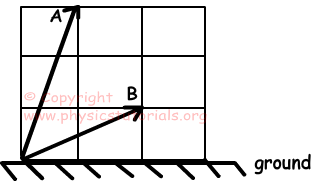Time of flight is directly proportional to vertical component of velocity. Vertical velocity component of A is three times bigger than vertical velocity component of B.

tA/tB=3       tB=tA/3

Horizontal distance traveled by the object is found by the following formula;

XA=VA.tA

XB=VB.tB

Horizontal component of VA is half of VB, so we can write following equation;

VA=VB/2

VB=2.VA

XA=VA.tA

XB=2.VA.tA/3

XA/XB=3/2

Author:

Tags: